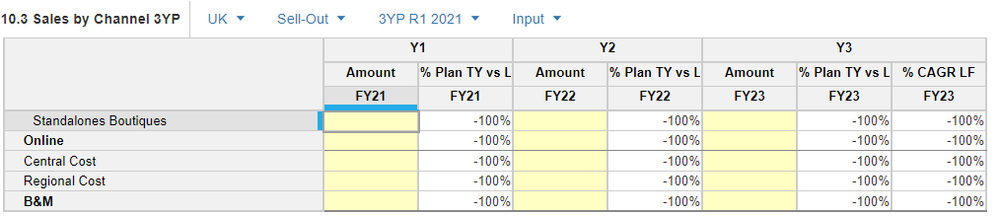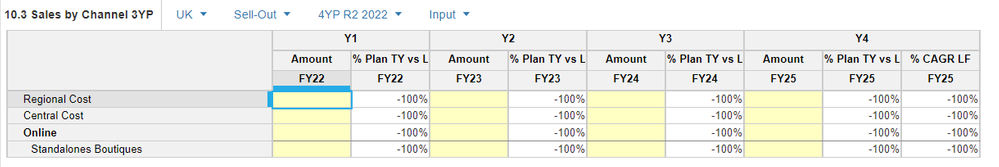# Initialize a specific dimension using another dimension from the same moduleHello

I currently have the following problem :

All the modules that constitute my model are run by a dimension "3 year Planning scenarios". This dimension is a list of different planning scenarios, each one has a different year of start as a setting ( initialized in a unique administration module)  . What i'd like for my model to have is the ability to add a new planning scenario (let's say for the following year) and initialize this scenario using a different scenario.

So if let's say i have a scenario starting in 2020 and extending on 2020,2021 and 2022. I'd like to make a process that creates a 2021 scenario as a member of the scenarios list, then initializes it on all of my modules by importing data from the 2020 scenario ( Here we would take the data for 2021 and 2022 , leaving 2023 empty )

Is there any way i could realisticly accomplish this ? If so where should i start ?

•@MehdiCH

Not sure if I understood it.

”This dimension is a list of” what does this mean?

Misbah

•Sorry if i havent phrased my question correctly, perhaps i should have been more clear.

Here is an exemple of of one the modules : you can see the dimension Planning scenarios which consists of 3YP 2021 or 4YP 2022 in this example. My question is how do i go about creating a process or actions that initializes a new member of these scenarios, using the data from other scenarios. Let's say for the 3YP 2021,i would like to create a new member called 3YP 2022, and import the data for that specific item using  data already input in the 3YP 2021. ( we would take 2022 and 2023, leaving 2024 empty)

Thanks for the answer, hope this clears things out